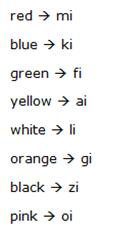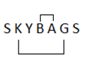# IBPS SO Prelims Reasoning Ability Questions 2019 (Day-1)

Dear Aspirants, Our IBPS Guide team is providing new series of Reasoning Questions for IBPS SO Prelims 2019 so the aspirants can practice it on a daily basis. These questions are framed by our skilled experts after understanding your needs thoroughly. Aspirants can practice these new series questions daily to familiarize with the exact exam pattern and make your preparation effective.

[WpProQuiz 7455]

Ensure Your Ability Before of Exam

Take IBPS SO Prelims Free Mock test

Coding Decoding

Directions (1 – 5): Study the following information to answer the given questions:

In a certain coded language,

‘red blue green yellow’ is written as ‘ki mi ai fi’

‘blue white orange green’ is written as ‘li fi gi ki’

‘green orange yellow black’ is written as ‘gi ai zi fi’

‘red black pink white’ is written as ‘oi mi li zi’

1) How ‘pink’ is written in the given coded language?

a) gi

b) oi

c) zi

d) Can’t be determined

e) None of these

2) How ‘red black saffron’ is written in the given coded language?

a) mi zi oi

b) zi li ri

c) fi oi zi

d) ei zi mi

e) None of these

3) Code ‘ki gi ri oi’ stands for which of the following?

a) white orange green saffron

b) orange saffron pink blue

c) orange pink saffron white

d) white pink saffron blue

e) None of these

4) What is the code for ‘blue black’ in the given coded language?

a) ki li

b) ki fi

c) li zi

d) mi zi

e) None of these

5) What is the code for ‘yellow’ in the given coded language?

a) ai

b) ri

c) li

d) mi

e) None of these

Blood relation

Directions (6 – 8): Study the following information carefully and answer the given questions:

Mahesh starts walking from A towards south direction for 2 km to reach point B. Then he takes a left turn and walks 4 km till C. From C he takes a left turn walks for2 km towards D. From D he takes a right turn and walks 6km till E. Finally, he moves towards north-east direction at an angle of 450and walks for 7 km till point F.

6) How far and in which direction is point A from E?

a) 17km, west

b) 10km, east

c) 10km, west

d) 2km north

e) None of these

7) What is the shortest distance between C and E?

a) 10√2 km

b) 5√2 km

c) 3 km

d) 2√10 km

e) None of these

8) Mahesh house is 10km north-west at an angle of 450 of point E then what is the distance between Mahesh house and Point C?

a) 10km

b) 12km

c) 8km

d) Can’t be determined

e) None of these

Miscellaneous

9) In a class of 45, where girls are twice that of boys, Rakesh ranked fourteenth from the top, if there are 10 girls ahead of Rakesh. How many boys are after him in rank?

a) 12

b) 09

c) 10

d) 11

e) None of these

10) How many letters are there in between the letters of the word “SKYBAGS” as many as are there in alphabetical series (both backward and forward)?

a) none

b) one

c) three

d) two

e) None of these

Directions (1-5):Directions (6-8):x2 = 22 + 62 = 4 + 36 = 40

=> x = √(4 * 10) = 2√10

DE = 6; E (Mahesh House) = 10 then D(Mahesh House) = √(102 – 62) = √64 = 8km + 2 km (distance between D and C) = 10 km

No of boys = x; No of girls = 2x;

x+2x = 45 => 3x = 45

x (Boys)= 15 ; 2x(Girls) = 30

Number of student behind Rakesh = 45 – 14 = 31

No of girls behind Rakesh = 30 – 10 = 20

No of boys behind Rakesh = 31 – 20 = 11